Scan QR code or get instant email to install app

Question:

# 1212If x > 0 and y > 0, which of the following expressions is equivalent to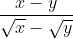?

A 1212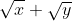explanation

1212Since x > 0 and y > 0, x can be rewritten as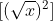and y can be rewritten as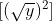. It follows, then, thatcan be rewritten as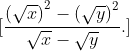Because the numerator is a difference of two squares, it can be factored as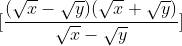. Finally, dividing the common factors of $(\sqrt x - \sqrt y )$ in the numerator and denominator yields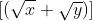.